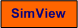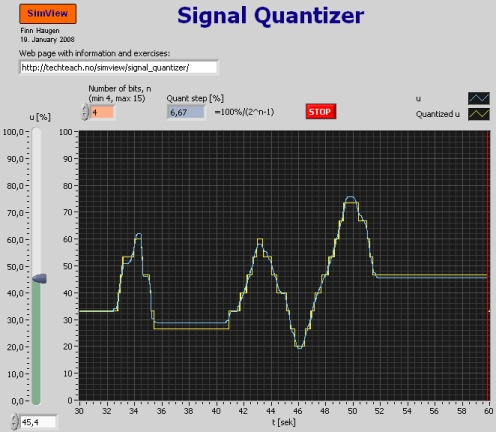# Signal Quantizer

Snapshot of the front panel of the simulator:• What is needed to run the the simulator? Read to get most recent information!
• Tips for using the simulator.
• The simulator: . The simulator runs immediately after the download by clicking Open in the download window. Alternatively, you can first save a copy of the exe-file on any directory (folder) on your PC and then run the exe-file, which starts the simulator.

## Description of the simulated system

In this simulator the sampling of an analog, continuous-time signal by an AD converter (analog-digital) is simulated.

When an analog, continuous-time signal y(t) of signal range [Ymin, Ymax] is sampled by an AD (analog-digital) converter of n bits: bn-1, bn,..., b1, b0, the resolution, i.e. the maximum signal range represented by the LSB (least significant bit), is

q = (Ymax - Ymin)/(2n - 1)

Example: Given a signal y between Ymax = 100% and Ymin = 0% which is sampled using a 8 bit AD converter. The resolution is

q = (Ymax - Ymin)/(2n - 1)
= (100% - 0%)/(28 - 1)
= 100%/255
= 0.392 %

## Aim

The aim of the tasks below is to give an understanding of how the sampled (discrete-time signal) depends on the number of bits in the AD converter.

## Motivation

Sampling av continuous-time signals takes place in all applications where a computer is used to read measurement data. It is important to be able to calculate the resolution of the digital signal generated by the AD converter to decide if the accuracy is acceptable.